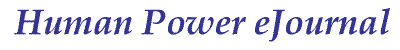article 09, issue 02

# Analysis and Visualisation of Data Collected from Human-Electric Hybrid Bicycle-Tests

Andreas Fuchs
October 15, 2005

Abstract

The German non-profit organization ExtraEnergy tested human-electric hybrid vehicles  in 2001 and in 2002, (e-bikes and pedelecs, see note).  This paper compiles, visualizes and discusses the resulting data. Such compilations are helpful to benchmark new vehicles or drive systems, to show what levels of performance are common and  to identify what kind of products are best suited for the user's intended application.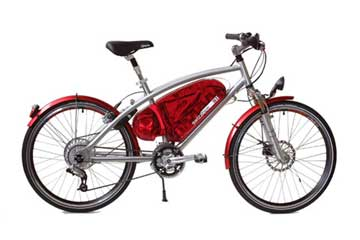The Dolphin is an example of a commercially produced electric bike

### Test Methods

A detailed description of these tests appears in ExtraEnergy's test reports  and .  Both trial series took place in realistic field conditions with many different riders. The tests were conducted both on mainly level and on mainly constant slope courses. On a "mixed track" portion of the testing, the riders stopped several times to simulate red lights.  The distance vehicles were able to travel (range) was determined by riding until the batteries were considered discharged.

Differences between both tests were:

1. In the 2001 test the assist ratio of electric power to human power wasn't measured.  Climbing speed was recorded on two different ascending grades; one had  an average 6.3% slope (max 8%), the other exhibited an average 8% slope (max 12%).  Range on slopes was not recorded.
2. In the 2002 test the assist-ratio was measured by comparing the power assisted vehicles with similar unassisted bicycles.  All vehicles were equipped with force-measuring pedals.  Power (product of pedaling torque and cadence) was measured at three different speeds of the unassisted reference bike and for all assisted bikes. Electrical power used by the system was measured at the battery.

### Test Data

The format in which the test data was reported was different in 2001 and 2002. For easier analysis most of the data of both tests was compiled into a table. See Appendix.

In 2002 the amount of power assist was reported using the assist rate (R) and the assist factor (F). The assist rate is the ratio of the electric drive power (Pe), measured at the battery to the total mechanical power delivered to the drive wheel (Pw). The assist factor is the ratio of electric drive power (Pe) to the human supplied power as measured at the pedals (Php).  If the assist factor is greater than 1, then the electric power is greater than the human's power contribution.

The assist rate and factor are derived as follows:

Php = Human power (pedalling power)
Pe = Power of the electric drive
Pw = Total drive power at wheel
R = Assist rate = Pe / Pw
F = Assist factor = Pe / Php = R / (1-R)

Because the human energy contribution (Php) is measured at the pedals instead of at the drive wheel, these relationships assume a pedal drive efficiency of 100% whereas the efficiency of the motor drive is unknown. [This means that the assist factor measured here is higher than the actual effective assist factor. Ed.]

These factors can be used to estimate the contributions of human power and electric power to range (at the same speeds):

Range using electric power only = total range * assist rate
Range using human power only = total range - range using electric power only

### Analysis

Drive Performance and Energy Expenditure

It was found that most parameters hardly differed between the two tests.

However, in 2002 the average battery capacity was higher than in 2001: 193 Wh instead of 155 Wh.

Bike prices didn't vary much within the same classes. The average price, excepting the fast Swiss bikes, was about 1500 Euro.

Key results for both 2001 and 2002 were:

• About 30 km range and 20 km/h average speed on level/mixed-track
• About 7 km range at a speed of 11 km/h on slope of 7% [about 500 m altitude gain, i.e. total range back to starting altitude would be at least 14 km, with higher average speed. Ed.]

 In detail: x Average Standard Deviation Min Max 2001 & 2002 Vehicle mass (kg) 29.1 5.6 18.1 42.5 Battery mass (kg) 5.9 3.2 2.2 15.6 Range on the level (km) 29.9 8.3 15.0 49.6 Speed on the level (km/h) 20.2 2.5 15.9 24.6 2002 only Range on slope 7% (km) 7.3 2.3 3.7 11.6 Speed on slope 7% (km/h) 10.8 2.1 7.7 13.8
[Table 1] The highest variation (standard deviation divided by average) is found in weight of battery. Average speed varies relatively little.[Figure 1] Ranges and average speeds of the tested bikes on the level. The average of ranges was about 30km and the average of average speeds was about 20 km/h. The top speed of most vehicles was (by law) under 24km/h in hybrid mode; that of the Dolphin 39 km/h.

Dividing range by battery capacity (see Appendix) gives energy use (including human power but not considering this in the calculation):

• Energy use is about 0.18 km/Wh or 6 Wh/km on the level
• Energy use is about 0.04 km/Wh or 27 Wh/km on the slopes

Using the assist rate (calculated from data in Appendix) one can easily estimate how far the human electric hybrids would have gone using only electric power (at the same speed): (Range * Assist rate) = Range using electric power only. The "purely electric range" would average 16.5 km and the energy use would average 12.4 Wh/km on the level.

If it is assumed that all tested bikes would have the same aerodynamic drag (effective frontal area assumed to be 0.5 m2) and rolling resistance (coefficient of rolling resistance assumed to be 0.007), then the ratio of the power needed to go on the level and to climb can be estimated. On the 7% slope at 11 km/h the total power required is about 2.5 times larger than on the level at 20 km/h. The ratio of energy use measured above is however 27 / 6 = 4.5. This is probably because at higher power levels the actual (in)efficiency of the electric drive weighs more strongly.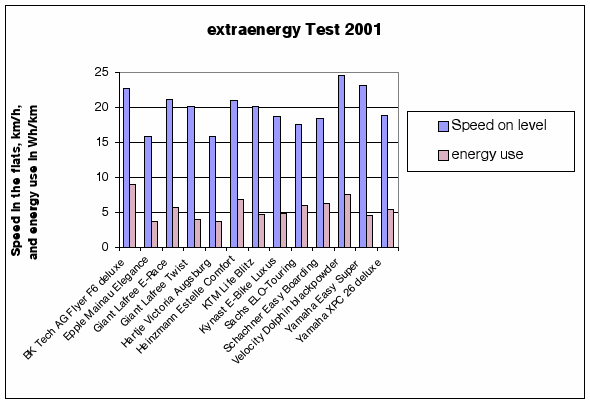[Figure 2] Average speed and energy use for various models.

This shows that in general higher average speed leads to higher energy use (Wh/km) when riding on the level, but with considerable variations.[Figure 3] Assist factor on the level and on the slope for various models.

On average, the assistance does not change much from the level to the slope: on the level the assist factor is 1.23, and on the slopes 1.77. These ratios are higher than the 1 : 1 factor much promoted and discussed in the 1990's.

These figures also show that while in many cases the assist factors do not differ much between level and slope, those of vehicles made in the hilly and alpine regions of Europe have much higher assist factors on slopes than on the level.

### Dependencies between Parameters

By plotting parameters against others one can try to identify those most important for the performance.

We plotted the "performance parameters" like range and speed over the "feature parameters" like price, weight of vehicle, weight of battery, assist factors in order to identify trends that could exist. Here are some results of interest.

We would like to know the answers to questions like: "Do the lighter or the heavier bikes climb better?" "Does range on the level change much with average speed ?"

In the graphs below, a linear regression line with an upward slope shows a positive correlation. The figure R2 gives the degree correlation between the parameters, from 0 (no correlation) to 1 (strong correlation).[Figure 4] Speed on slope vs. battery capacity.[Figure 5] Speed on slope vs. assist factor.

 Other (mainly expected) correlations: Year of Test Parameter 2 Parameter 1 Slope R2 2001 Max. speed Price 0.007 km/h/Euro 0.72 2001 Speed on slope Battery capacity 0.04 km/h/Wh 0.38 2001 Speed on slope Mass of Bike 0.32 km/h/kg 0.36 Both years Range on the level Battery capacity 0.074 km/Wh 0.29 2002 Speed on the level Assist factor 1.94 km/h/[-] 0.24 2002 Range on the level Assist factor 6.5 km/[-] 0.19 Both years Energy use on the level Speed on the level 0.3 Wh/km/km/h 0.17
[Table 2] Examples of some stronger correlations between features and performance of vehicles

Examples for unexpectedly weak correlations:

• Energy use on slope [Wh/km] versus assist factor [no units]
• Energy use on slope [Wh/km] versus speed [km/h]

The vehicles in the 2001 test climbed faster the heavier they were, since the available power depends on the mass of the motors and batteries, in contrast to unassisted bikes, where the opposite is true. [This implies an optimum configuration for each hybrid operating point. Ed.]

Additionally, if batteries are dimensioned too small, the discharge efficiency is low at high power levels and therefore the range on slopes decreases.

### Visualisation

The data may also be used to visualize general facts for individual vehicles or average values.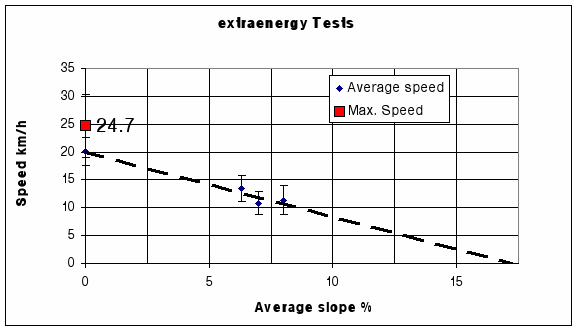[Figure 6] Averages of average speed vs. average slope.

Average speed on the level is about 20 km/h (all samples), the maximum average speed 24.7 km/h (Velocity Dolphin). [Personal experience with the Dolphin also shows a much flatter curve. Ed.]

At 10 % slope the tested vehicles go nearly 10 km/h.

The dashed line is an extrapolation and suggests a maximum climbing slope of 18%. Exact values would depend on the actual gearing.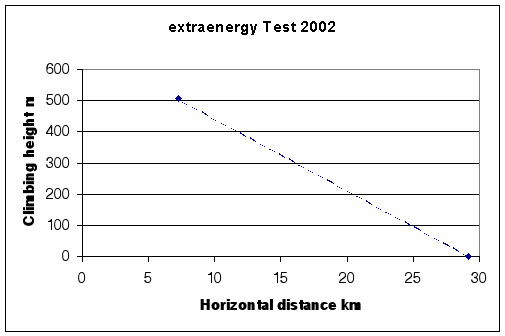[Figure 7] Average climbing height vs. horizontal distance.

Such graphs in sales literature would help consumers decide on the suitability of vehicles for the planned usage.

An e-bike may be defined as a bicycle equipped with an auxiliary electrical motor which operates independently from human power input.  A pedelec (pedal electric) is a vehicle equipped with an auxiliary electric motor that operates only when the rider pedals. A legal definition of a pedelec at: ExtraEnergy.org.

### Top of PageReferences:

 2001 Test, Extra Energy e.V., Testbericht.pdf

 Pedelec / E-Bike-Test 2002, Extra Energy e.V., TestberichtRaeder.pdf

### Appendix:

Spreadsheet of 2001 and 2002 tabulated results; public access (zipped XML) format (appendix.sxc 11 KB) or proprietary format (appendix.xls 16KB)